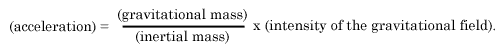Albert Einstein: Relativity
Part II: The General Theory of Relativity

### The Gravitational Field

"If we pick up a stone and then let it go, why does it fall to the ground ?" The usual answer to this question is: "Because it is attracted by the earth." Modern physics formulates the answer rather differently for the following reason. As a result of the more careful study of electromagnetic phenomena, we have come to regard action at a distance as a process impossible without the intervention of some intermediary medium. If, for instance, a magnet attracts a piece of iron, we cannot be content to regard this as meaning that the magnet acts directly on the iron through the intermediate empty space, but we are constrained to imagine — after the manner of Faraday — that the magnet always calls into being something physically real in the space around it, that something being what we call a "magnetic field." In its turn this magnetic field operates on the piece of iron, so that the latter strives to move towards the magnet. We shall not discuss here the justification for this incidental conception, which is indeed a somewhat arbitrary one. We shall only mention that with its aid electromagnetic phenomena can be theoretically represented much more satisfactorily than without it, and this applies particularly to the transmission of electromagnetic waves. The effects of gravitation also are regarded in an analogous manner.

The action of the earth on the stone takes place indirectly. The earth produces in its surrounding a gravitational field, which acts on the stone and produces its motion of fall. As we know from experience, the intensity of the action on a body dimishes according to a quite definite law, as we proceed farther and farther away from the earth. From our point of view this means : The law governing the properties of the gravitational field in space must be a perfectly definite one, in order correctly to represent the diminution of gravitational action with the distance from operative bodies. It is something like this: The body (e.g. the earth) produces a field in its immediate neighbourhood directly; the intensity and direction of the field at points farther removed from the body are thence determined by the law which governs the properties in space of the gravitational fields themselves.

In contrast to electric and magnetic fields, the gravitational field exhibits a most remarkable property, which is of fundamental importance for what follows. Bodies which are moving under the sole influence of a gravitational field receive an acceleration, which does not in the least depend either on the material or on the physical state of the body. For instance, a piece of lead and a piece of wood fall in exactly the same manner in a gravitational field (in vacuo), when they start off from rest or with the same initial velocity. This law, which holds most accurately, can be expressed in a different form in the light of the following consideration.

According to Newton's law of motion, we have

(Force) = (inertial mass) x (acceleration),

where the "inertial mass" is a characteristic constant of the accelerated body. If now gravitation is the cause of the acceleration, we then have

(Force) = (gravitational mass) x (intensity of the gravitational field),

where the "gravitational mass" is likewise a characteristic constant for the body. From these two relations follows:If now, as we find from experience, the acceleration is to be independent of the nature and the condition of the body and always the same for a given gravitational field, then the ratio of the gravitational to the inertial mass must likewise be the same for all bodies. By a suitable choice of units we can thus make this ratio equal to unity. We then have the following law: The gravitational mass of a body is equal to its inertial law.

It is true that this important law had hitherto been recorded in mechanics, but it had not been interpreted. A satisfactory interpretation can be obtained only if we recognise the following fact : The same quality of a body manifests itself according to circumstances as " inertia " or as " weight " (lit. " heaviness '). In the following section we shall show to what extent this is actually the case, and how this question is connected with the general postulate of relativity.